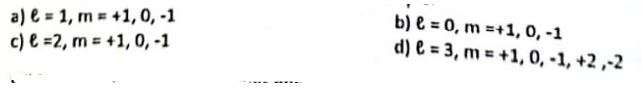# Chemistry Practice MCQs Chapter 5 Class 11

581
Created on By Ali Durrani

Chemistry Ch 5 Class 11

1 / 20

If an election jumps from 6th orbit to 2nd orbit then decrease in radius will be?

2 / 20

What are the values of orbital quantum number/0 and magnetic quantum number(m) for subshell?

3 / 20

Number of transitions possible for an electron in the 6th shell are

4 / 20

If an electron jumps from fourth to first orbit, which of the following series will it show?

5 / 20

Bracket series of 14-atom is in which of the following regions of electromagnetic spectrum?

6 / 20

When alpha particles are bombarded on beryllium

7 / 20

d subshell has

8 / 20

Which quantum number decides energy of orbitals?

9 / 20

Which of the following quantum numbers are for p-subshell?10 / 20

In a discharge tube, at room pressure there is

11 / 20

Which orbitals always possess same energy:

12 / 20

Spectrum of which series is in the UV region?

13 / 20

Spectrum of which series is in the visible region?

14 / 20

) The velocity of electron in outermost shell Is?

15 / 20

P.E of an electron in the orbit of an atom Is

16 / 20

Breaking of neutron produces?

17 / 20

If an electron of hydrogen atom jumps from 5th orbit to 7’h orbit, its atomic radius increases

18 / 20

If an electron makes a transition from 4th orbit to inner orbits, then the wavelength for which the radius is maximum, lies in which of the following series?

19 / 20

Diameter of Hydrogen atom is?

20 / 20

The correct order of orbitals is: